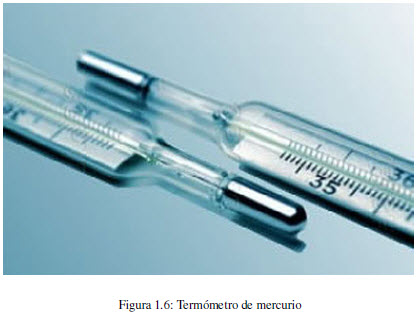Heat is a form of energy transfer, which occurs by virtue of a temperature difference. The flow of heat always occurs from the hot body to the cold.

The temperature of a body can be measured with mercury thermometers, which consist of a reservoir for mercury attached to a capillary. When the tank is heated, the mercury expands through the capillary. The higher the temperature, the higher the mercury rise is observed.

In order to measure temperatures, it is necessary to have a temperature scale. Swedish astronomer Anders Celsius developed the so-called Celsius temperature scale. He takes as points of reference the melting of water, to which he assigns 0º Celsius, and its boiling point at atmospheric pressure, to which he assigns 100º Celsius. Between these points there are one hundred divisions, each representing a degree Celsius.

A widely used temperature scale in the United States is Fahrenheit. On this scale the freezing and boiling points of water are taken to be 32ºF and 212ºF.

In the scientific world, temperatures are expressed on the kelvin scale. This scale takes the lowest temperature that can exist as zero, which is why it is called the absolute temperature scale.

The relationships between the different temperature scales are given by the following mathematical equations: